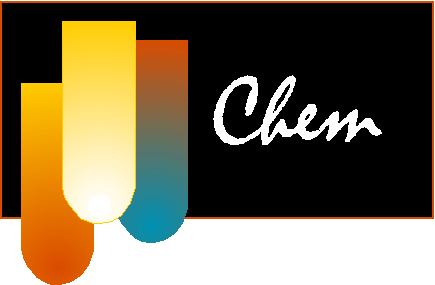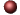Gases & Kinetic Molecular TheoryExamples ofMultiple Choice Questions

1.
Which statement is false?
(a) The density of a gas is constant as long as its temperature remains constant.
(b) Gases can be expanded without limit.
(c) Gases diffuse into each other and mix almost immediately when put into the same container.
(d) The molecular weight of a gaseous compound is a non-variable quantity.
(e) Pressure must be exerted on a sample of a gas in order to confine it.

2.
A sample of oxygen occupies 47.2 liters under a pressure of 1240 torr at 25oC. What volume would it occupy at 25oC if the pressure were decreased to 730 torr?
(a) 27.8 L
(b) 29.3 L
(c) 32.3 L
(d) 47.8 L
(e) 80.2 L

3.
A sample of nitrogen occupies 5.50 liters under a pressure of 900 torr at 25oC. At what temperature will it occupy 10.0 liters at the same pressure?
(a) 32oC
(b) -109oC
(c) 154oC
(d) 269oC
(e) 370oC

4.
Under conditions of fixed temperature and amount of gas, Boyle's law requires that
I. P1V1 = P2V2
II. PV = constant
III. P1/P2 = V2/V1
(a) I only
(b) II only
(c) III only
(d) I, II, and III
(e) another combination

5.
The volume of a sample of nitrogen is 6.00 liters at 35oC and 740 torr. What volume will it occupy at STP?
(a) 6.59 L
(b) 5.46 L
(c) 6.95 L
(d) 5.67 L
(e) 5.18 L

6.
The density of chlorine gas at STP, in grams per liter, is approximately:
(a) 6.2
(b) 3.2
(c) 3.9
(d) 4.5
(e) 1.3

7.
What pressure (in atm) would be exerted by 76 g of fluorine gas in a 1.50 liter vessel at -37oC?
(a) 26 atm
(b) 4.1 atm
(c) 19,600 atm
(d) 84
(e) 8.2 atm

8.
What is the density of ammonia gas at 2.00 atm pressure and a temperature of 25.0oC?
(a) 0.720 g/L
(b) 0.980 g/L
(c) 1.39 g/L
(d) 16.6 g/L
(e) 0.695 g/L

9.
A container with volume 71.9 mL contains water vapor at a pressure of 10.4 atm and a temperature of 465oC. How many grams of the gas are in the container?
(a) 0.421 g
(b) 0.183 g
(c) 0.129 g
(d) 0.363 g
(e) 0.222 g

10.
What is the molecular weight of a pure gaseous compound having a density of 4.95 g/L at -35 oC and 1020 torr?
(a) 24
(b) 11
(c) 72
(d) 120
(e) 44

11.
A 0.580 g sample of a compound containing only carbon and hydrogen contains 0.480 g of carbon and 0.100 g of hydrogen. At STP, 33.6 mL of the gas has a mass of 0.087 g. What is the molecular (true) formula for the compound?
(a) CH3
(b) C2H6
(c) C2H5
(d) C4H10
(e) C4H12

12.
A mixture of 90.0 grams of CH4 and 10.0 grams of argon has a pressure of 250 torr under conditions of constant temperature and volume. The partial pressure of CH4 in torr is:
(a) 143
(b) 100
(c) 10.7
(d) 239
(e) 26.6

13.
What pressure (in atm) would be exerted by a mixture of 1.4 g of nitrogen gas and 4.8 g of oxygen gas in a 200 mL container at 57oC?
(a) 4.7
(b) 34
(c) 47
(d) 27
(e) 0.030

14.
A sample of hydrogen gas collected by displacement of water occupied 30.0 mL at 24oC on a day when the barometric pressure was 736 torr. What volume would the hydrogen occupy if it were dry and at STP? The vapor pressure of water at 24.0oC is 22.4 torr.
(a) 32.4 mL
(b) 21.6 mL
(c) 36.8 mL
(d) 25.9 mL
(e) 27.6 mL

15.
Which one of the following statements is not consistent with the kinetic-molecular theory of gases?
(a) Individual gas molecules are relatively far apart.
(b) The actual volume of the gas molecules themselves is very small compared to the volume occupied by the gas at ordinary temperatures and pressures.
(c) The average kinetic energies of different gases are different at the same temperature.
(d) There is no net gain or loss of the total kinetic (translational) energy in collisions between gas molecules.
(e) The theory explains most of the observed behavior of gases at ordinary temperatures and pressures.

16.
A mixture of 0.50 mol H2(g) and 0.50 mol N2(g) is introduced into a 15.0 liter container having a pinhole leak at 30oC. After a period of time, which of the following is true?

(a) The partial pressure of H2 exceeds that of N2 in the container.
(b) The partial pressure of N2 exceeds that of H2 in the container.
(c) The partial pressures of the two gases remain equal.
(d) The partial pressures of both gases increase above their initial values.
(e) The partial pressure of H2 in the container increases above the initial value.

17.
If helium effuses through a porous barrier at a rate of 4.0 moles per minute, at what rate (in moles per minute) would oxygen gas diffuse?
(a) 0.20
(b) 0.50
(c) 2.0
(d) 8.0
(e) 1.41

18.
A real gas most closely approaches the behavior of an ideal gas under conditions of:
(a) high P and low T
(b) low P and high T
(c) low P and T
(d) high P and T
(e) STP

19.
Which one of the following statements about the following reaction is false?
CH4(g) + 2O2(g)CO2(g) + 2H2O(g)
(a) Every methane molecule that reacts produces two water molecules.
(b) If 32.0 g of oxygen reacts with excess methane, the maximum amount of carbon dioxide produced will be 22.0 g.
(c) If 11.2 liters of methane react with an excess of oxygen, the volume of carbon dioxide produced at STP is (44/16)(11.2) liters.
(d) If 16.0 g of methane react with 64.0 g of oxygen, the combined masses of the products will be 80.0 g.
(e) If 22.4 liters of methane at STP react with 64.0 g of oxygen, 22.4 liters of carbon dioxide at STP can be produced.

20.
What total gas volume (in liters) at 520oC and 880 torr would result from the decomposition of 33 g of potassium bicarbonate according to the equation:
2KHCO3(s)K2CO3(s) + CO2(g) + H2O(g)
(a) 56 L
(b) 37 L
(c) 10 L
(d) 19 L
(e) 12 L

21.
Calculate the weight of KClO3 that would be required to produce 29.5 L of oxygen measured at 127oC and 760 torr.
2KClO3(s)2KCl(s) + 3O2(g)
(a) 7.82 g
(b) 12.2 g
(c) 14.6 g
(d) 24.4 g
(e) 73.5 g

22.
Which of the following statements is false?
(a) The properties of N2(g) will deviate more from ideality at -100oC than at 100oC.
(b) Van der Waal's equation corrects for the non-ideality of real gases.
(c) Molecules of CH4(g) at high pressures and low temperatures have no attractive forces between each other.
(d) Molecules of an ideal gas are assumed to have no significant volume.
(e) Real gases do not always obey the ideal gas laws.

23.
The ideal gas law predicts that the molar volume (volume of one mole) of gas equals:
(a) gRT/PV
(b) (MW)P/RT
(c) 1/2ms-2
(d) RT/P
(e) 22.4 L at any temperature and pressure

24.
Three 1.0 liter flasks are filled with H2, O2 and Ne, respectively, at STP.
Which of the following statements is true?

(a) Each flask has the same number of gas molecules.
(b) The velocity of the gas molecules is the same in each flask.
(c) The density of each gas is the same.
(d) There are twice as many O2 and H2 molecules as Ne atoms.
(e) None of the above is true.

25.
For a gas, which pair of variables are inversely proportional to each other (if all other conditions remain constant)?
(a) P, T
(b) P, V
(c) V, T
(d) n, V
(e) n, P

1. (a) 2. (e) 3. (d) 4. (d) 5. (e) 6. (b) 7. (a) 8. (c) 9. (e) 10. (c) 11. (d) 12. (d) 13. (d) 14. (d) 15. (c) 16. (b) 17. (e) 18. (b) 19. (c) 20. (d) 21. (e) 22. (c) 23. (d) 24. (a) 25. (b)Click here to return to the top.Choose your next chapter:
Fundamentals of Chemistry | Chemical Formulas & Composition Stoichiometry | Chemical Equations & Rxn Stoichiometry | Types of Chemical Reactions |
| Atomic Structure | Chemical Periodicity | Chemical Bonding | Molecular Structure/Covalent Bonding Theories| Molecular Orbital Theory |
| Acids/Bases/Salts - Theory & Rxns | Acids/Bases/Salts - Calculations (including balancing redox rxns) | Gases | Solids & Liquids | Solutions |
| Thermodynamics | Kinetics | Equilibrium | Aqueous Equilibrium - Acids/Bases/Salts | Aqueous Equilibrium - Buffers & Titrations |
| Aqueous Equilibrium - Slightly Soluble Salts | Electrochemistry | Metallurgy | Metal Properties & Rxns | Nonmetals & Metalloids |
| Coordination Compounds | Nuclear Chemistry | Organic Chem - Formulas/Names/Properties | Organic Chem - Shapes/Rxns/Biopolymers |To report any corrections, please e-mail Dr. Wendy Keeney-Kennicutt.